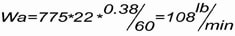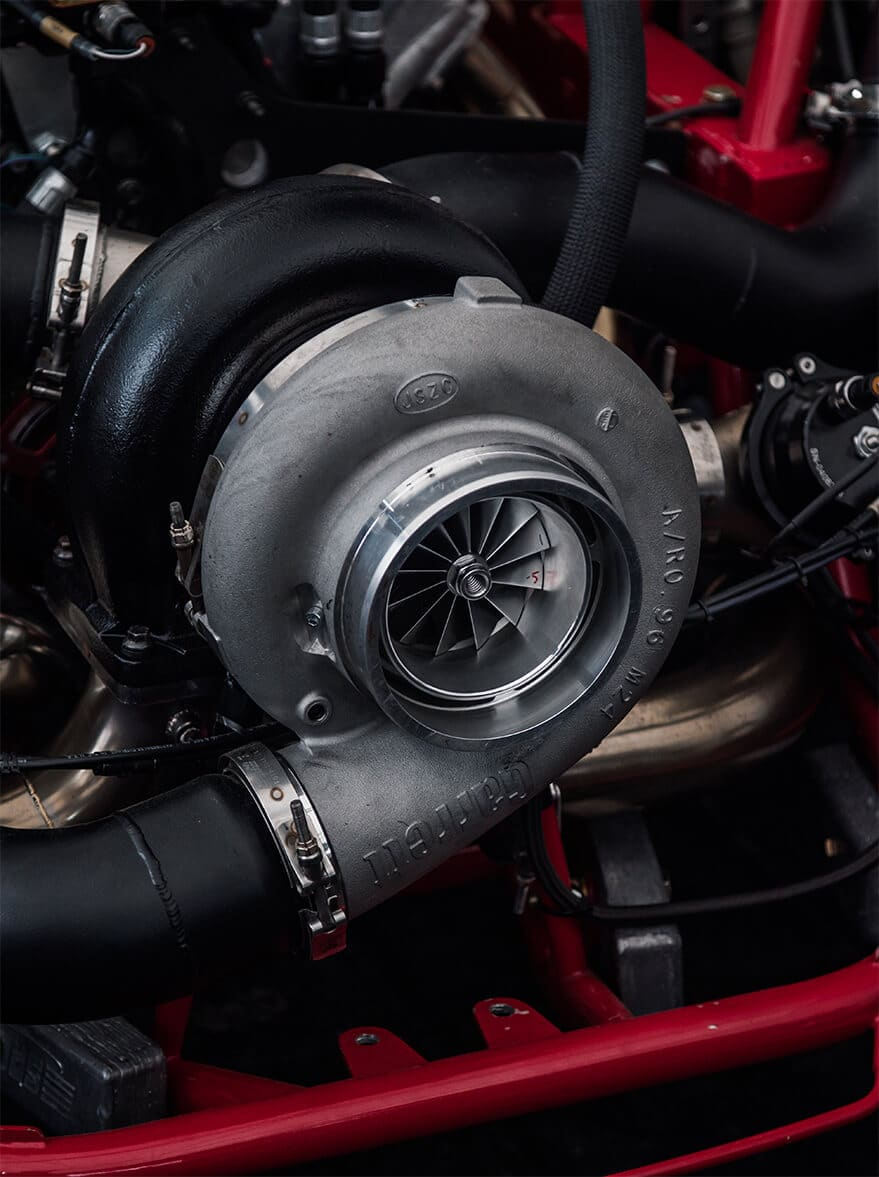# 选择合适的涡轮增压器

## 增压顾问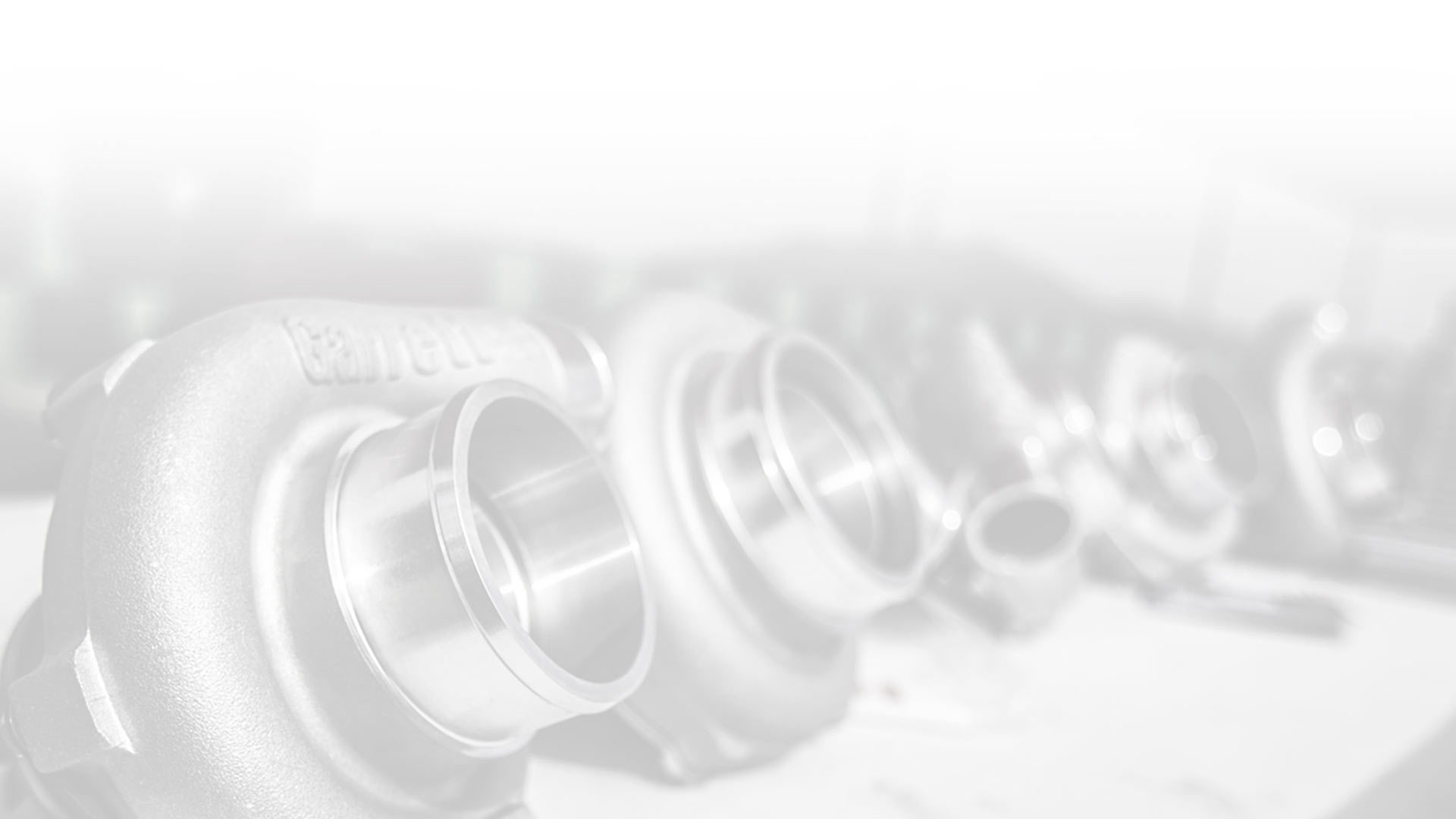## 选择合适的柴油升级涡轮增压器需要了解什么？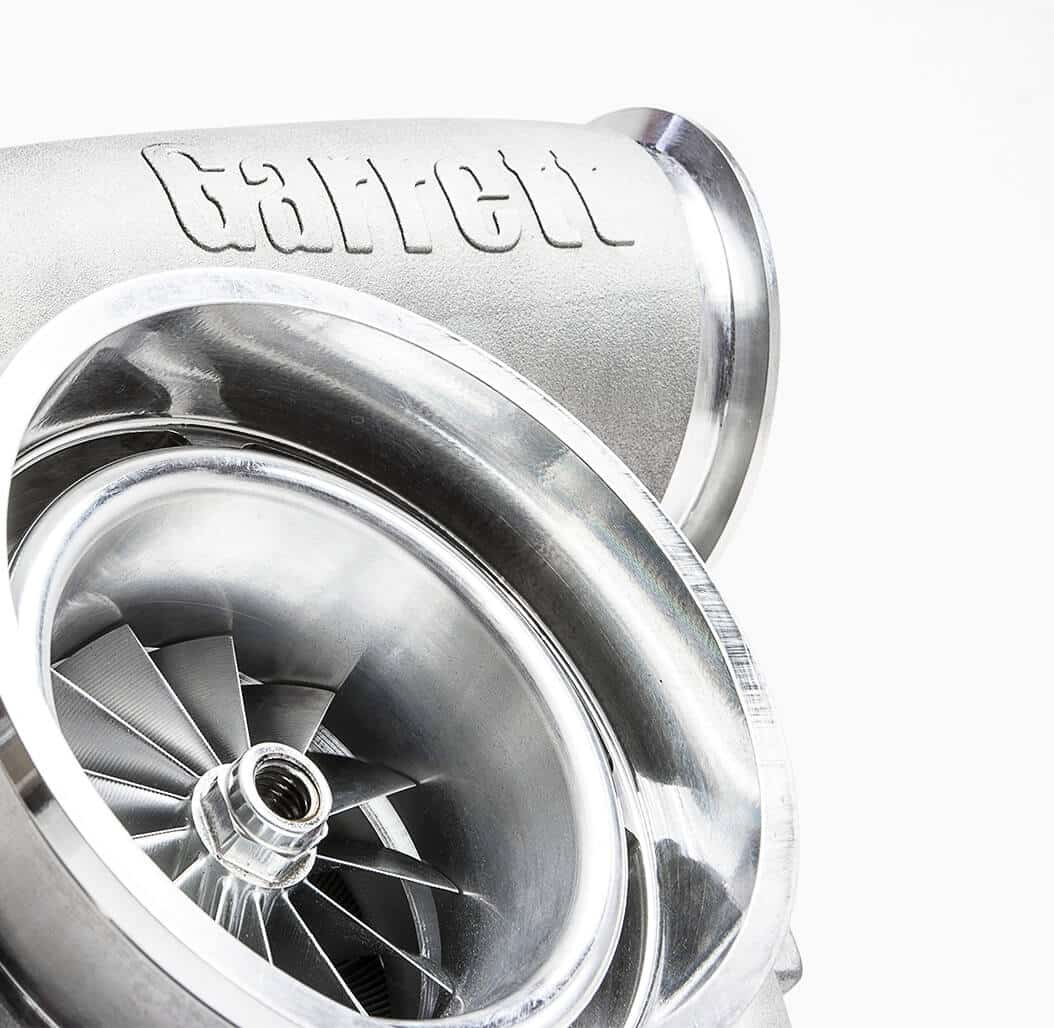## 太棒了，那么我该选择哪种涡轮增压器？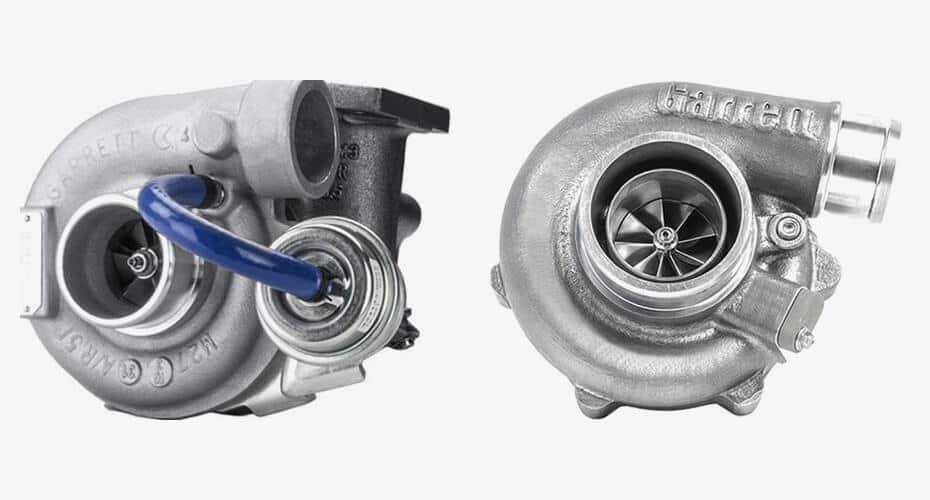## 示例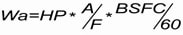HP=马力目标值（飞轮）
A/F=空燃比
BSFC/60=比油耗（lb/（Hp*hr））/60（从小时转换为分钟）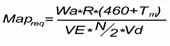MAPreq=满足马力目标值所需的歧管绝对压力（psia）
Wa=Airflowactual（lb/min）
R=气体常数=639.6
Tm =进气歧管温度（°F）
VE =容积效率
N=发动机转速（RPM）
Vd=发动机排量（立方英寸，通过乘以61，从升转换为CI，例如2.0升* 61 = 122 CI）

Wa =先前计算的59.2 lb/min
Tm = 130°F
VE=98%
N=3300 RPM
Vd = 6.6升*61 = 400 CI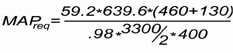= 34.5 psia（记住，这是绝对压力;减去大气压力以获得表压，34.5 psia – 14.7 psia（海平面）= 19.8 psig）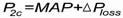P2c =压缩机排气压力（psia）
MAP =歧管绝对压力（psia）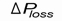=压缩机和歧管之间的压力损失（psi）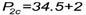=36.5 psia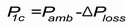•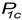=压缩机入口压力（psia）
•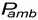=环境空气压力（psia）
•=空气过滤器/管道（psi）造成的压力损失
•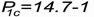=13.7 psia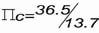=2.7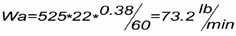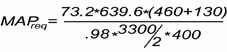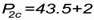=45.5 psia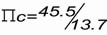=3.3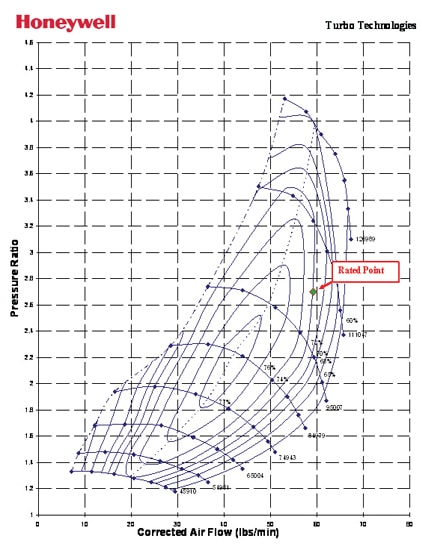(从技术上来说，发动机可能很容易利用以前的压缩机来制造该动力，但是它有冒烟更多、EGT和背压更高的风险；有点像使现有压缩机超出使用极限…)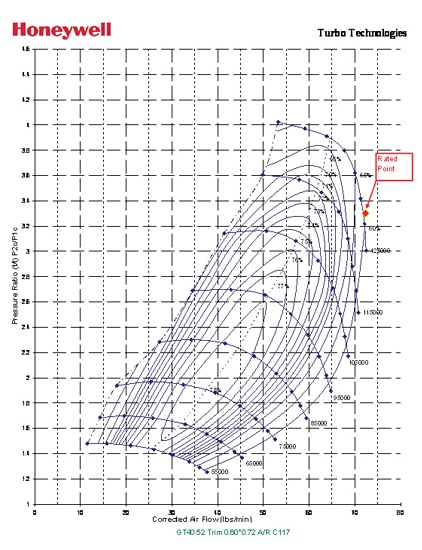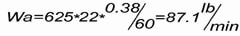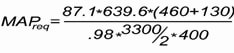=50.8 psia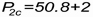=52.8 psia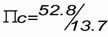=3.8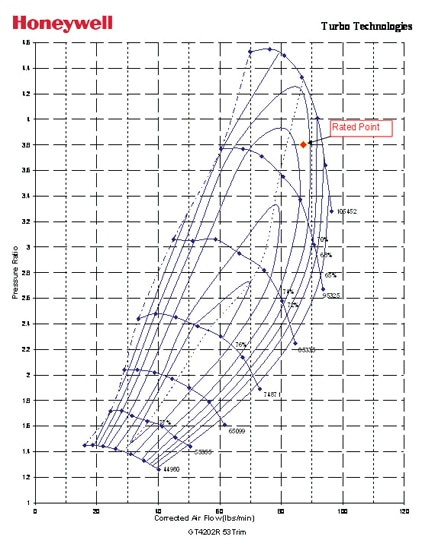## 最后案例：竞赛类别